# Density method

(diff) ← Older revision | Latest revision (diff) | Newer revision → (diff)

One of the methods in analytic number theory based on the study of statistics of the distribution of the zeros of the Riemann zeta-function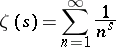and Dirichlet-functions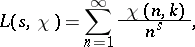where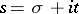andis a character modulo. Many number-theoretic problems obtain their final solution on the assumption that all the zeros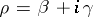of the functions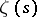and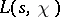in the strip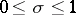,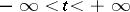lie on the straight line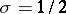. However, in some cases, sufficiently strong results are obtained if one can show that the zeros of these functions with abscissas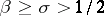, if they exist, constitute a set that becomes more sparse as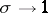. There are numerous theorems that give upper bounds for the number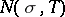of zeros ofand for the number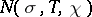of zeros ofin the rectangle,. The density method is substantially based on these theorems, which have been called density theorems.

G. Hoheisel (1930) was the first to use the density method with density theorems forin order to estimate the difference between two adjacent prime numbers. He was able to show that there is a positive constant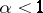such that for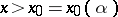there is always a prime number betweenand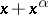. Subsequently, any improvement in the bound forled to a more precise constant. Yu.V. Linnik (1944 and subsequent years) developed the density method for Dirichlet-functions; he was the first to examine the distributions of the zeros of-functions for variable, and in particular obtained results on the "frequency" of the zeros ofnear the point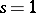, which provided a bound for the least prime number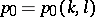in the arithmetic progression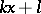,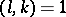,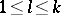,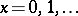: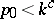, whereis some absolute constant. Improving the bounds onleads to a more precise constant. Linnik, in applying density theorems for-functions, derived a new proof of Vinogradov's theorem on the representation of any sufficiently large odd number as the sum of three prime numbers (see Goldbach problem).

The density method in the theory of-functions has provided a strong result in the binary Goldbach problem: Any sufficiently large natural number can be represented as the sum of two prime numbers and some power of two bounded by an absolute constant.

The strongest results from the density method are obtained in combination with other methods, in particular with the method of the large sieve. The Vinogradov–Bombieri theorem (1965), which in many cases replaces the generalized Riemann hypothesis (cf. Riemann hypothesis, generalized) was proved in this way. The ideas and results from the density method can be transferred from the field of rational numbers to an algebraic number field.

How to Cite This Entry:
Density method. Encyclopedia of Mathematics. URL: http://encyclopediaofmath.org/index.php?title=Density_method&oldid=18483
This article was adapted from an original article by B.M. Bredikhin (originator), which appeared in Encyclopedia of Mathematics - ISBN 1402006098. See original article